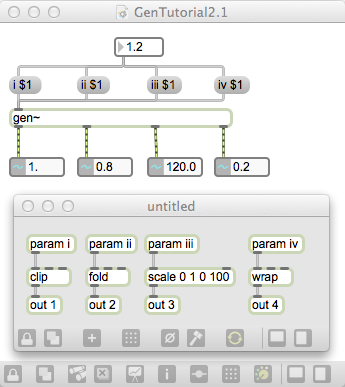Yesterday we created our own [+~] and a simple crossfader in Gen. Today we are going to look at more Gen operators to build a simple delay object. Gen contains Mathematical (+, -, * etc.), Logical (!, &&), Comparative (==, >), and range and routing operators as found in Max. Some very useful, but potentially confusing, operators are clip, fold, scale, and wrap.

• scale works very similar to [scale] and [scale~] by scaling an input range (i.e. 0, 1) to an output range (i.e. 0, 100). Note there is no clamping!
• clip clamps the input between min and max (i.e. 0, 1), so an input of 1.2 would be clamped to 1.0.
• fold folds the input between min and max (i.e. 0, 1), so an input of 1.2 would be folded down to 0.8.
• wrap wraps the input between min and max (i.e. 0, 1), so an input of 1.2 would be wrapped to 0.2.

To understand these better, play around with the following patch.Gen also contains built in dsp operators such as cycle, buffer, pashor etc. So, using cycle, we can create our own sinetone generator.Operators common to the audio and visual domains can be found here, and audio only [gen~] operators can be found here.

Remember that data in Gen is always synchronous, and hence a feedback loop isn’t possible. Thus two very useful operators are delay and history. history is a single-sample delay where any input triggers the output of the previous input. Multiple history operators can be daisy-chained to create longer delays, but this is more efficiently achieved using delay which delays a signal by a certain amount of time, specified in samples.

Lets create a simple delay patch.So we create a one-second delay (44100 samples) where the audio folds between [-1, 1]. A feedback is created by mixing this delayed sample with the previous feedback value. A delayFeedback param controls this from Max, with the current feedback value stored in history for the next sample. This feedback value is then crossfaded with the original signal, controlled by delayDryWet param. Notice that both delayFeedback and delayDryWet are clamped to the range [0, 1] and given initial values.